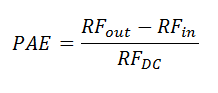# Power Aided Efficiency Calculator

This CalcTown calculator calculates the power-added frequency (PAE) of a RF amplifier with the efficiency equation. Enter the input power, output power and the DC power supplied to the amplifier to calculate the efficiency.

We know efficiency of the RF amplifier will help to analyze how much power is dissipated as heat. This is the parameter used to design heat sink for the RF amplifier. There are three main types of efficiency viz. power added efficiency, overall efficiency and drain efficiency.

#### Result

%Where,

RFin = Amplifier input power

RFout = Amplifier output power

RFDC = Amplifier DC power input

PAE = Power-added efficiency of the amplifier